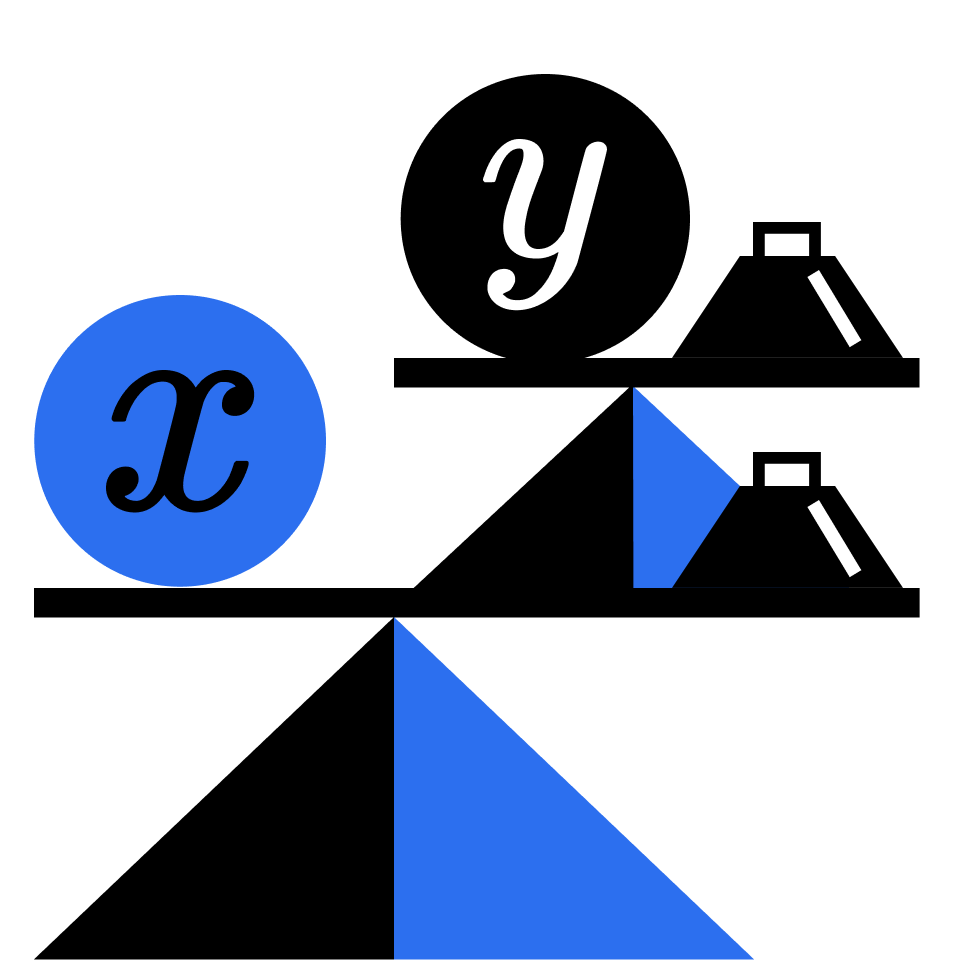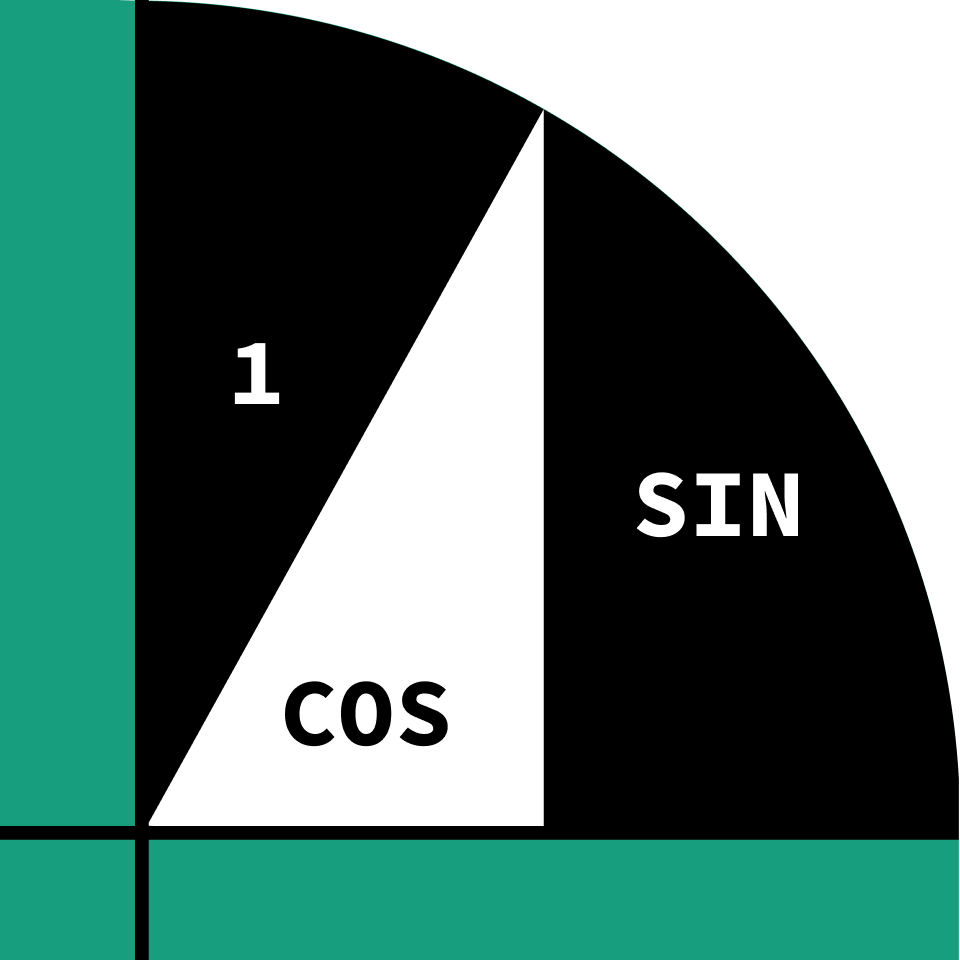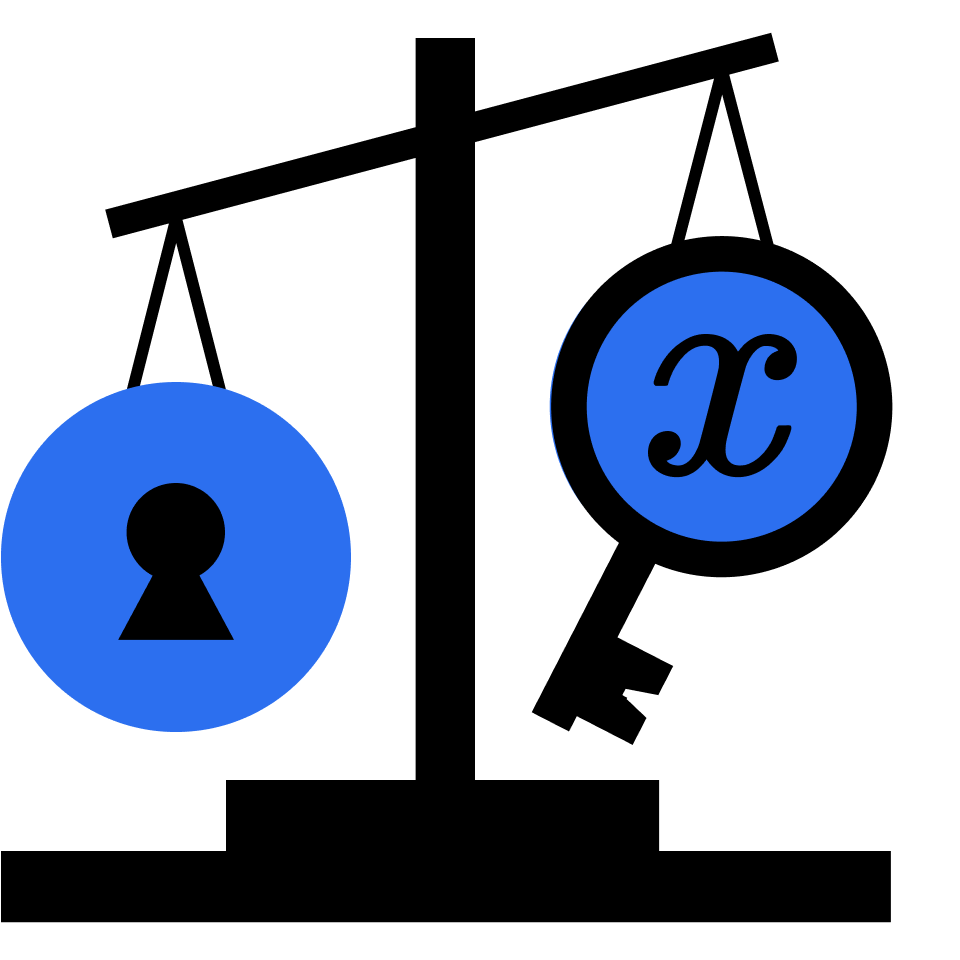# Interactive Courses to Learn Math

## Learn math from dozens of hands-on, interactive lessons in logic, probability, algebra, geometry, and more.

### Courses covered#### Algebra through Puzzles

• Arithmetic Sequences
• Algebra Shortcuts
• Balanced Scale Puzzles
• Calcdoku Puzzles
• Difference of Squares
• Fibonacci Numbers
• Geometric Sequences
• Inverse Square Laws
• Joint Proportionality
• Linear Equations
• Magic Squares
• Solving Systems of Equations#### Mathematical Fundamentals

• Algebraic Expressions
• Arithmetic Operators
• Cryptogram Puzzles
• Discount Finance
• Divisibility
• Divisibility Rules
• Logic Fundamentals
• Rates and Ratios
• Sequences
• The Distributive Property
• Variables#### Calculus Fundamentals

• Continuity
• Derivatives
• Extrema
• Implicit Derivatives
• L'Hôpital's Rule
• Limits
• Newton's Method
• Physics Applications
• The Chain Rule
• The Squeeze Theorem#### Geometry Fundamentals

• Angle Axioms
• Coordinate Geometry
• Invariant Areas
• Parallel and Perpendicular Lines
• Perimeter
• Similarity
• Surface Area
• The Pythagorean Theorem
• Three-Dimensional Figures
• Volume#### Pre-Algebra

• Bar Models
• Distributive Property
• Evaluating Expressions
• Factoring
• Factors
• Inverses
• Order of Operations
• Simplifying Expressions
• Solving Equations
• Variables#### Introduction to Probability

• Bayes' Rule
• Counting
• Distributions
• Expectation Value
• Independence
• Random Walks
• Rule of Product
• Rule of Sum
• Simulation
• Waiting Time

### Over 50,000 5-star reviews on iOS App Store and Google Play### See math and science in a new way

All of our courses are crafted by award-winning teachers, researchers, and professionals from MIT, Caltech, Duke, Microsoft, Google, and more.

#### Courses in Software Development

• Computer Science Fundamentals
• Algorithm Fundamentals
• Programming with Python
• Data Structures
• Introduction to Neural Networks
• Computational Biology